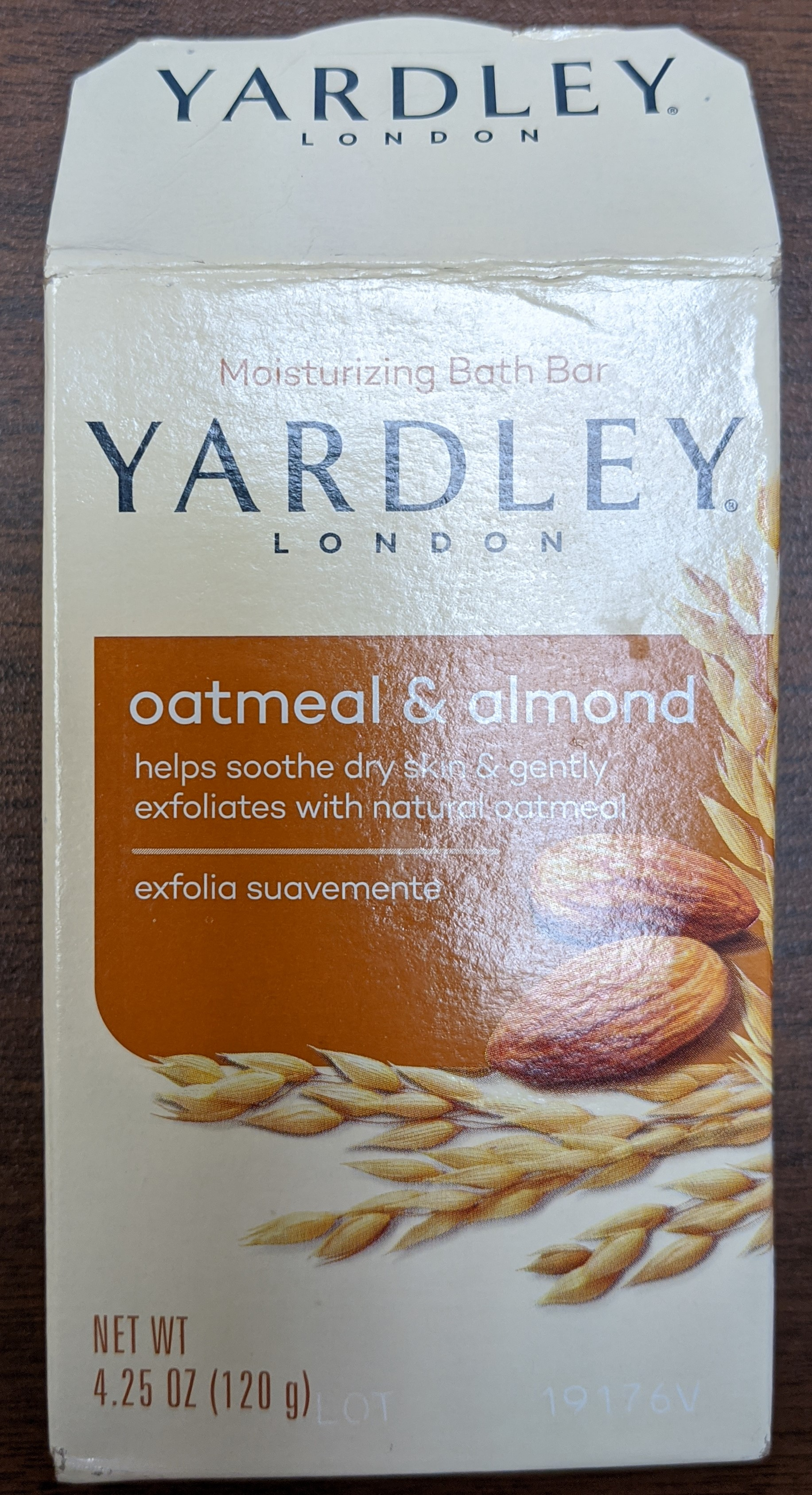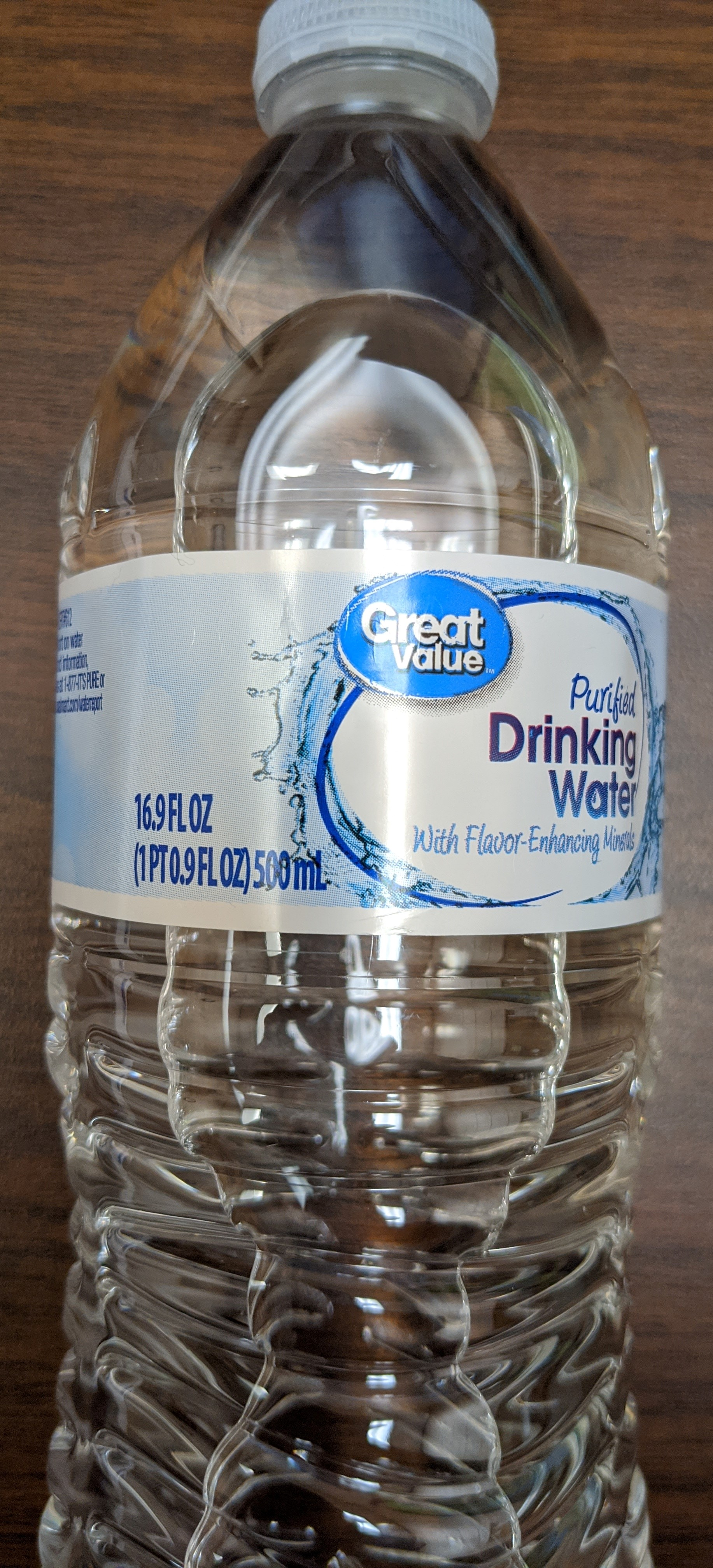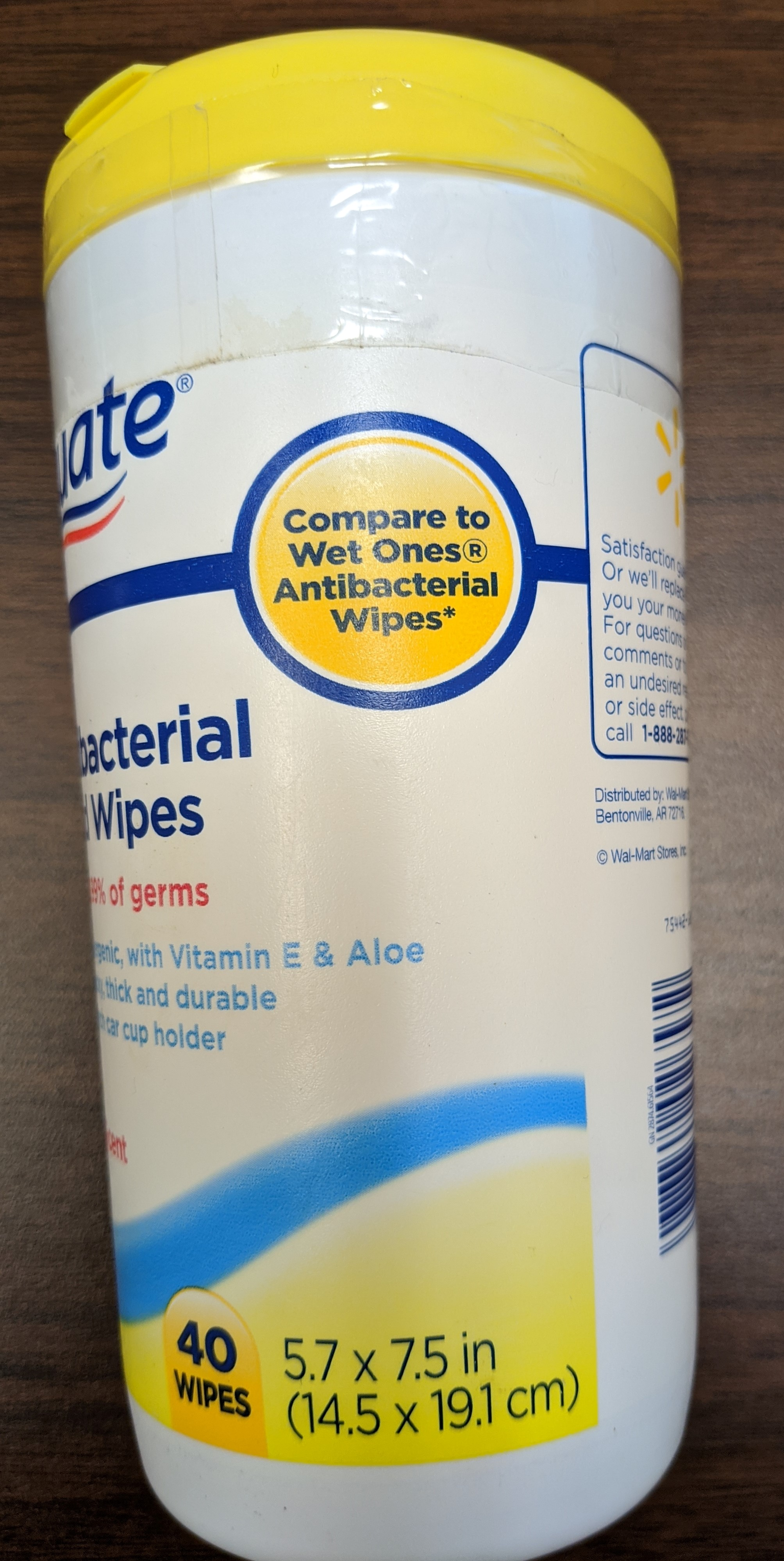### Los Angeles

We had the best time playing at Venice Beach!### New York

The atmosphere in New York is lorem ipsum.### Chicago

Thank you, Chicago - A night we won't forget.

For in GOD we live, and move, and have our being. - Acts 17:28

The Joy of a Teacher is the Success of his Students. - Samuel Dominic Chukwuemeka

# Measurements and UnitsI greet you this day,
Second: view the videos.
Third: solve the questions/solved examples.
Fourth: check your solutions with my thoroughly-explained solutions.
Fourth: check your solutions with the calculators. Please follow the directions specified in the Conversion from Any Unit to Any Unit
Comments, ideas, areas of improvement, questions, and constructive criticisms are welcome. You may contact me.
If you are my student, please do not contact me here. Contact me via the school's system.
Thank you for visiting!!!

Samuel Dominic Chukwuemeka (Samdom For Peace) B.Eng., A.A.T, M.Ed., M.S

## Objectives

Students will:

(1.) Discuss Dimensional Analysis.

(2.) Discuss Fundamental Quantities.

(3.) Discuss Derived Quantities.

(4.) Discuss the International Metric System (SI) of units for measured quantities.

(5.) Discuss the United States Customary System of units for measured quantities.

(6.) Convert between units for linear measures.

(7.) Convert among units for linear measures.

(8.) Convert between units for quadratic measures/area measures.

(9.) Convert among units for quadratic measures/area measures.

(10.) Convert between units for cubic measures/volume measures.

(11.) Convert among units for cubic measures/volume measures.

(12.) Convert between International Metric System of units and United States Customary System of units.

(13.) Perform arithmetic operations of quantities in the International Metric System.

(14.) Perform arithmetic operations of quantities in the United States Customary System.

(15.) Solve applied problems on Measurements and Units.

(16.) Complete a student project on Measurements and Units.

Skills Measured/Acquired
(1.) Use of prior knowledge
(2.) Critical Thinking
(3.) Interdisciplinary connections/applications
(4.) Technology
(5.) Active participation through direct questioning
(6.) Research

## Vocabulary Words

quantity, unit, fundamental quantity, derived quantity, dimensional analysis, dimension, measurement, international system of unit, metric system of unit, international metric system, united states system of unit, customary system of unit, united states customary system, yotta, zetta, exa, peta, tera, giga, mega, kilo, hecto, deka, yocto, zepto, atto, femto, pico, nano, micro, milli, centi, deci,

## General Project Requirements

(1.) This is an individual project.
It is not a group project.
Students may work together. However, each student must submit his/her own project.

(2.) The items only allowed in the project include containers of:
(a.) Food
(b.) Drinks (excluding alcoholic drinks)
(c.) Soaps
(d.) Lotion
(e.) Paint
If you are unsure whether any container is permitted, please ask the Professor accordingly.

(3.) The image of the container used must be included.
According to your course syllabus, attachments are not allowed.
Any attachment submitted will not be opened nor graded.
Please use the Insert/Edit Image icon to insert the image directly.
The image should be clear.

(4.) Please use only the 3 tables given to you.
Any use of any other table will lead to deduction of points.
The only $3$ tables are:

Metric to Metric Conversions
Prefix Symbol Multiplication Factor
yocto y $10^{-24}$
zepto z $10^{-21}$
atto a $10^{-18}$
femto f $10^{-15}$
pico p $10^{-12}$
nano n $10^{-9}$
micro $\mu$ $10^{-6}$
milli m $10^{-3}$
centi c $10^{-2}$
deci d $10^{-1}$
deka da $10^1$
hecto h $10^2$
kilo K $10^3$
mega M $10^6$
giga G $10^9$
tera T $10^{12}$
peta P $10^{15}$
exa E $10^{18}$
zetta Z $10^{21}$
yotta Y $10^{24}$

Customary to Customary Conversions
Measurement Customary Customary Unit Conversion Factor
Length inch (in) foot (ft) $12\:inches = 1\:ft$
Length foot (ft) yard (yd) $3\:ft = 1\:yd$
Length yard (yd) mile (mi) $1760\:yd = 1\:mi$
Length foot (ft) mile (mi) $5280\:ft = 1\:mi$
Mass pound (lb) ounce (oz) $1\:lb = 16\:oz$
Mass short ton (ton) pound (lb) $1\:short\:ton = 2000\:lb$
Mass long ton pound (lb) $1\:long\:ton = 2240\:lb$
Mass stone pound (lb) $1\:\:stone = 14\:lb$
Mass long ton stone $1\:long\:ton = 160\:stones$
Area acre (acre) square feet ($ft^2$) $1\:acre = 43560\:ft^2$
Volume quart (qt) pint (pt) $1\:qt = 2\:pt$
Volume pint (pt) cup (cup) $1\:pt = 2\:cups$
Volume quart (qt) cup (cup) $1\:qt = 4\:cups$
Volume quart (qt) fluid ounce (fl. oz) $1\:qt = 32\:fl.\:oz$
Volume pint (pt) fluid ounce (fl. oz) $1\:pt = 16\:fl.\:oz$
Volume cup (cup) fluid ounce (fl. oz) $1\:cup = 8\:fl.\:oz$
Volume gallon (gal) quart (qt) $1\:gal = 4\:qt$
Volume gallon (gal) quart (pt) $1\:gal = 8\:pt$
Volume gallon (gal) cup (cup) $1\:gal = 16\:cups$
Volume gallon (gal) fluid ounce (fl. oz) $1\:gal = 128\:fl.\:oz$
Volume gallon (gal) cubic inches ($in^3$) $1\:gal = 231\:in^3$

Metric to Customary Conversions
Measurement Metric Customary Unit Conversion Factor
Length meter (m) foot (ft) $1\:ft = 0.3048\:m$
Mass gram (g) pound (lb) $1\:lb = 453.59237\:g$
Mass metric ton (tonne) kilogram (kg) $1\:tonne = 1000\:kg$
Volume liter or cubic decimeters
(L or $dm^3$)
gallons (gal) $1\:L = 0.264\:gal$

(5.) All work must be shown.
Please use the Launch Math Editor icon to type fractions, equations, and formulas among others.

You may use any or a combination of the 3 methods taught/discussed:
(a.) First Method: Unity Fraction Method
(b.) Second Method: Proportional Reasoning Method
(c.) Third Method: Fast Proportional Reasoning Method

If you do not want to use any of these method, you are welcome to use any other appropriate method.

If the converted quantity on the container was rounded:
(b.) Please round accordingly to match the converted quantity on the container.
(c.) Please specify the type of rounding that was done (how many decimal places, how many significant digits, etc)

(7.) For this project, you do not need to respond to the initial post of any student.
But, you are welcome to respond to any student's posts or responses.
Communication and collaboration is encouraged.
However, please note that responding to the initial post of any student will not yield any credit.
In other words, there is no extra credit for any extra work.

(8.) Please review the examples I did.
This will give you an idea of what I expect.
These are the minimum expectations.
I would like you to be more creative.
You may not do the same examples that I did.

(9.) All work must be turned in by the due date to receive credit.
Late work will not be accepted.

### Examples

NOTE: Unless specified otherwise:
(1.) Please do not approximate intermediate calculations.
(2.) Please do not approximate final calculations.

#### Example 1

Project: Measurements and Units
Objective: To convert a measurement from a unit to another unit.
Measurement: Mass
Given Unit: Customary unit (Ounce)
To Convert to: Metric unit (Gram)
Container Used: Soap
Please look at the bottom left corner.Convert $4.25\:oz$ to $g$

From Given Tables:

$1\:lb = 16\:oz \\[3ex] 1\:lb = 453.59237\:g \\[3ex] 1\:lb = 1\:lb \\[3ex] \implies 16\:oz = 453.59237\:g \\[3ex] Let\:\:p = mass\:\:of\:\:4.25\:oz\:\:in\:\:g \\[3ex] \underline{Second\:\:Method:\:\:Proportional\:\:Reasoning\:\:Method} \\[3ex]$

$oz$ $g$
$16$ $453.59237$
$4.25$ $p$

$\dfrac{p}{4.25} = \dfrac{453.59237}{16} \\[5ex] Multiply\:\:both\:\:sides\:\:by\:\:4.25 \\[3ex] 4.25 * \dfrac{p}{4.25} = 4.25 * \dfrac{453.59237}{16} \\[5ex] p = \dfrac{4.25 * 453.59237}{16} \\[5ex] p = \dfrac{1927.76757}{16} \\[5ex] p = 120.485473 \\[3ex] p \approx 120\:g...rounded\:\:to\:\:the\:\:nearest\:\:whole\:\:number \\[3ex] \therefore 4.25\:oz \approx 120\:g \\[3ex]$ This confirms the quantity in $g$ (in parenthesis) in the soap container.

#### Example 2

Project: Measurements and Units
Objective: To convert a measurement from a unit to another unit.
Measurement: Volume
Given Unit: Customary unit (Fluid Ounce)
To Convert to: Metric unit (Milliliters)
Container Used: Water
Please look at the top center corner.Convert $16.9\:fl\:\:oz$ to $mL$

From Given Tables:

$1\:L = 0.264\:gal \\[3ex] 1\:gal = 4\:qt \\[3ex] 1\:qt = 4\:cups \\[3ex] 1\:cup = 8\:fl.\:oz \\[3ex]$ Based on what we were given:
Let us first convert it to liters ($L$)
Then, we will convert from liters ($L$) to milliliters ($mL$)

$\underline{First\:\:Method:\:\:Unity\:\:Fraction\:\:Method} \\[3ex] 16.9\:fl\:oz\:\:to\:\:L \\[3ex] Set\:\:it\:\:up\:\:and\:\:check\:\:to\:\:make\:\:sure\:\:it\:\:is\:\:correct \\[3ex] 16.9\:fl\:oz * \dfrac{.....L}{.....gal} * \dfrac{.....gal}{.....qt} * \dfrac{.....qt}{.....cup} * \dfrac{.....cup}{.....fl.\:oz} \\[5ex] 16.9\:fl\:oz * \dfrac{1\:L}{0.264\:gal} * \dfrac{1\:gal}{4\:qt} * \dfrac{1\:qt}{4\:cup} * \dfrac{1\:cup}{8\:fl.\:oz} \\[5ex] = \dfrac{16.9 * 1 * 1 * 1 * 1}{0.264 * 4 * 4 * 8} \\[5ex] = \dfrac{16.9}{33.792} \\[5ex] = 0.500118371\:L \\[3ex] Convert\:\:0.500118371\:L\:\:to\:\:mL \\[3ex] \underline{First\:\:Method:\:\:Unity\:\:Fraction\:\:Method} \\[3ex] Set\:\:it\:\:up\:\:and\:\:check\:\:to\:\:make\:\:sure\:\:it\:\:is\:\:correct \\[3ex] 0.500118371\:L * \dfrac{.....mL}{.....L} \\[5ex] = 0.500118371\:L * \dfrac{1\:mL}{10^{-3}\:L} \\[5ex] = 0.500118371\:L * \dfrac{1\:mL}{0.001\:L} \\[5ex] = 500.118371\:mL \\[3ex] 500.118371\:mL \approx 500\:mL \\[3ex]$ This confirms the quantity in $mL$ in the water container.

Student: Sir, you could have used the direct conversion from gallons to cups...
and bypass quarts

$16.9\:fl\:oz * \dfrac{.....L}{.....gal} * \dfrac{.....gal}{.....cup} * \dfrac{.....cup}{.....fl.\:oz} \\[5ex] 16.9\:fl\:oz * \dfrac{1\:L}{0.264\:gal} * \dfrac{1\:gal}{16\:cups} * \dfrac{1\:cup}{8\:fl.\:oz} \\[5ex]$ Teacher: That is right!
You are correct.
But, what if you were given a table that does not have that direct conversion?
Student: Then, I would use what I was given.
But, in this case; we were given that direct conversion.

#### Example 3

Project: Measurements and Units
Objective: To convert a measurement from a unit to another unit.
Measurement: Lengths (Width by Length)
Given Unit: Customary unit (in by in)
To Convert to: Metric unit (cm by cm)
Container Used: Hand Wipes
Please look at the bottom center corner.Convert $5.7\:in\:\:by\:\:7.5\:in$ to $cm\:\:by\:\:cm$

$Width = 5.7\:in \\[3ex] Length = 7.5\:in \\[3ex]$ From Given Tables:

$1\:ft = 0.3048\:m \\[3ex] 12\:inches = 1\:ft \\[3ex] 1\:ft = 12\:inches \\[3ex] 1\:ft = 1\:ft \\[3ex] \implies 0.3048\:m = 12\:inches \\[3ex]$ We shall use the First Method to convert the width.
We shall use the Second Method to convert the length.
Use any method(s) you prefer.

$\underline{First\:\:Method:\:\:Unity\:\:Fraction\:\:Method} \\[3ex] Convert\:\:the\:\:Width \\[3ex] 5.7\:in \:\:to\:\: cm \\[3ex] Set\:\:it\:\:up\:\:and\:\:check\:\:to\:\:make\:\:sure\:\:it\:\:is\:\:correct \\[3ex] 5.7\:in * \dfrac{.....m}{.....in} * \dfrac{.....cm}{.....m} \\[5ex] 5.7\:in * \dfrac{0.3048\:m}{12\:in} * \dfrac{1\:cm}{10^{-2}\:m} \\[5ex] = 5.7\:in * \dfrac{0.3048\:m}{12\:in} * \dfrac{1\:cm}{0.01\:m} \\[5ex] = \dfrac{5.7 * 0.3048 * 1}{12 * 0.01} \\[5ex] = \dfrac{1.73736}{0.12} \\[5ex] = 14.478\:cm \approx 14.5\:cm \\[3ex] \underline{Second\:\:Method:\:\:Proportional\:\:Reasoning\:\:Method} \\[3ex] Convert\:\:the\:\:Length \\[3ex] Let\:\:p = length\:\:of\:\:7.5\:in\:\:in\:\:m \\[3ex] Let\:\:c = length\:\:of\:\:p\:m\:\:in\:\:cm \\[3ex]$ Based on what we were given:
We need to first convert to meters ($m$)
Then, we will convert from meters ($m$) to centimeters ($cm$)

From Given Tables:

$1\:ft = 0.3048\:m \\[3ex] 12\:inches = 1\:ft \\[3ex] 1\:ft = 12\:inches \\[3ex] 1\:ft = 1\:ft \\[3ex] \implies 0.3048\:m = 12\:inches \\[3ex] 1\:cm = 10^{-2}\:m \\[3ex] 1\:cm = 0.01\:m$

$in$ $m$
$12$ $0.3048$
$7.5$ $p$

$\dfrac{p}{7.5} = \dfrac{0.3048}{12} \\[5ex] Multiply\:\:both\:\:sides\:\:by\:\: 7.5 \\[3ex] 7.5 * \dfrac{p}{7.5} = 7.5 * \dfrac{0.3048}{12} \\[5ex] p = \dfrac{7.5(0.3048)}{12} \\[5ex] p = \dfrac{2.286}{12} \\[5ex] p = 0.1905\:m \\[3ex]$
$m$ $cm$
$0.01$ $1$
$0.1905$ $c$

$\dfrac{c}{1} = \dfrac{0.1905}{0.01} \\[5ex] c = 19.05\:cm \approx 19.1\:cm$

The results confirm the quantities in $cm$ in the hand wipe container.